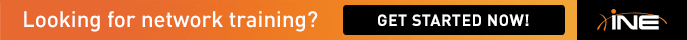# ospf cost calculations

Hi all

can ne one answer the following

hi mate

By ne chance on lab 3 do you know how they calculated the cost on task 4.6
it says cost = reference bw / interface bw

can you calculate the following

BW OSPF COST
10,000 2
10 2000
1,544 12953

If you can write out the formult in full for the 1st one wil be appreciated

thanks

• By default, the cost is calculated as:

Cost = Reference BW/Interface BW

The default Reference BW is 100Mb. In order to arrive at the costs you mention from the solution, you need to increase the Reference BW to 20,000Mb. So you get:

Cost = 20,000Mb/10Mb
Cost = 2000

When you enter the auto-cost reference-bandwidth command under the OSPF process, it expects the value in Mb:

Rack1R5(config-router)#auto-cost reference-bandwidth ?
<1-4294967> The reference bandwidth in terms of Mbits per second

Entering 20000 will then give you the requested costs.

Steve